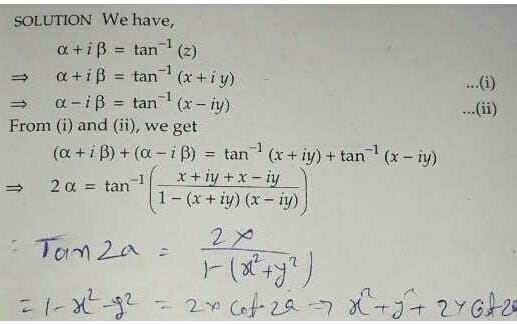If α + i β = tan⁻1 z, z = x ...
If α + i β = tan⁻1 z, z = x + yi and α is constant, then locus of \'z\' is
• a)
x2 + y2 + 2x cot 2α = 1
• b)
(cot 2α) (x2 + y2) = 1 + x
• c)
x2 + y2+ 2y tan 2α = 1
• d)
x2 + y2 + 2x sin 2α = 1
Correct answer is option 'A'. Can you explain this answer?

### Related TestPavan Patil May 20, 2020
Related If α + i β = tan⁻1 z, z = x + yi and α is constant, then locus of \'z\' isa)x2 + y2 + 2x cot 2α = 1b)(cot 2α) (x2 + y2) = 1 + xc)x2 + y2+ 2y tan 2α = 1d)x2 + y2 + 2x sin 2α = 1Correct answer is option 'A'. Can you explain this answer?1 Crore+ students have signed up on EduRev. Have you?Jananda Vardhan May 08, 2020
Correct answer is option a